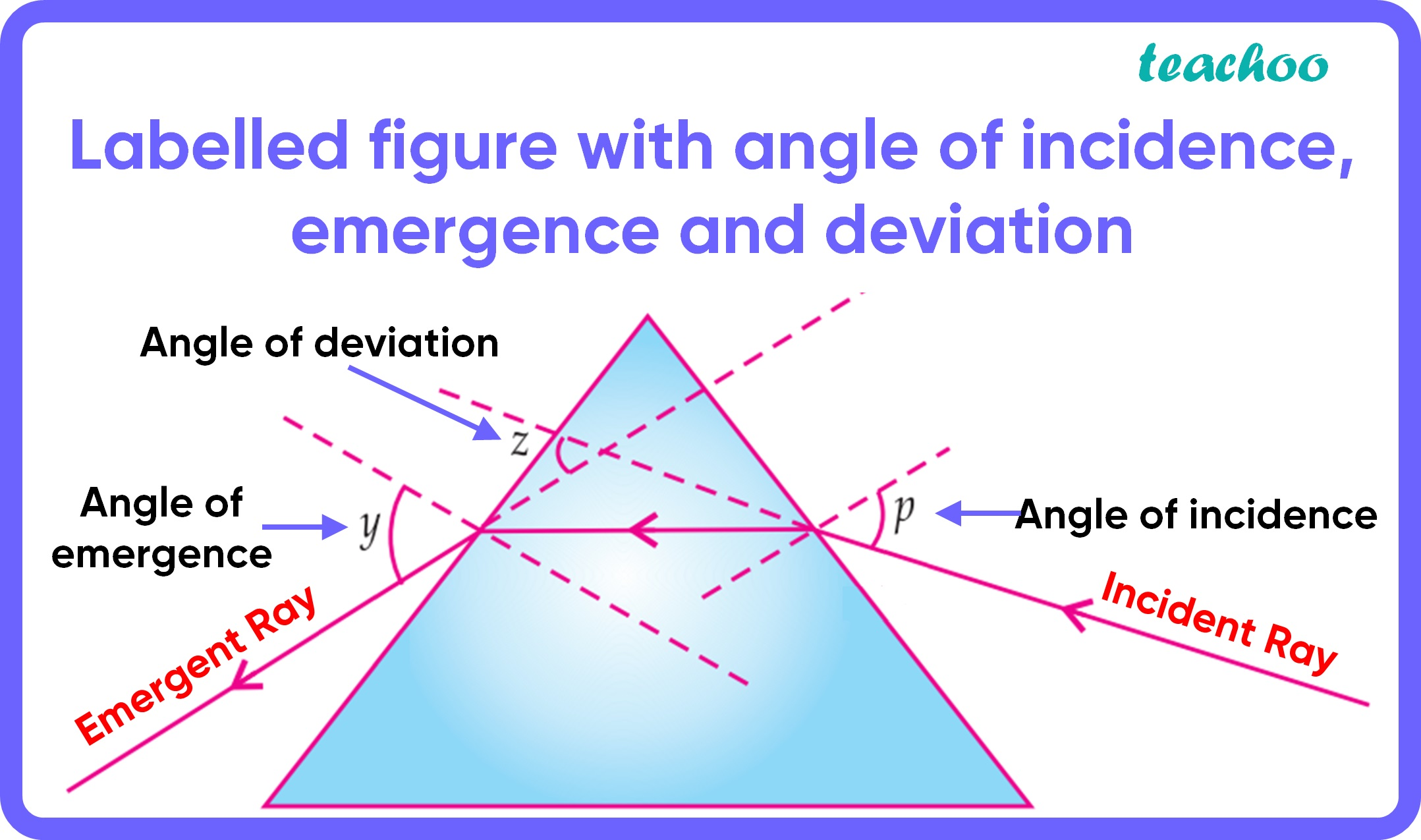MCQ from Past Year Papers

Class 10
Chapter 11 Class 10 - Human Eye and Colourful World

## (D) p, z and y

• The angle between the incident ray   and the normal is known as the angle of incidence .
This is angle y in our figure

• The angle between the emergent ray and the normal is known as the angle of emergence

This is angle y in our figure

• The angle between emergent ray and incident ray produced backwards is called the angle of deviation .So, the correct answer is (c) p, y and z

Get live Maths 1-on-1 Classs - Class 6 to 12